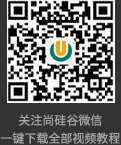# 三、使用消息队列来避免分布式事务

1． 如何可靠保存凭证（消息）

• 业务与消息耦合的方式

Begin transaction

update A set amount=amount-10000 where userId=1;

insert into message(userId, amount,status) values(1, 10000, 1);

End transaction

commit;

②业务与消息解耦方式

2.如何解决消息重复投递的问题

for each msg in queue

Begin transaction

select count(*) as cnt from message_apply where msg_id=msg.msg_id;

if cnt==0 then

update B set amount=amount+10000 where userId=1;

insert into message_apply(msg_id) values(msg.msg_id);

End transaction

commit;

# 相关推荐